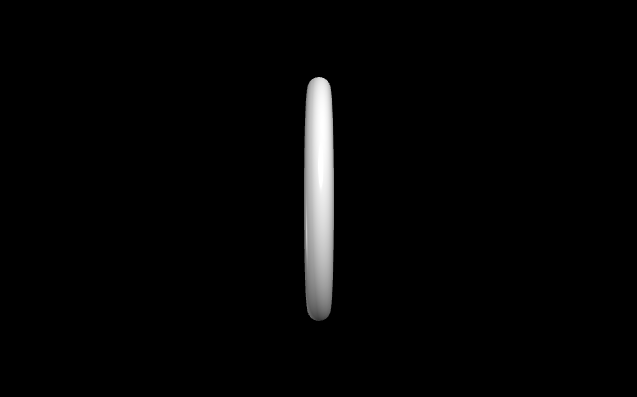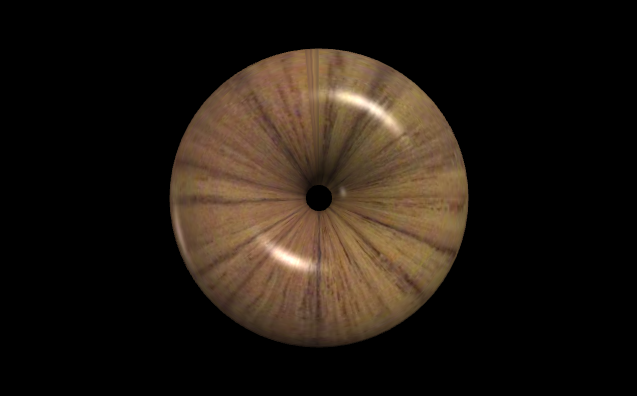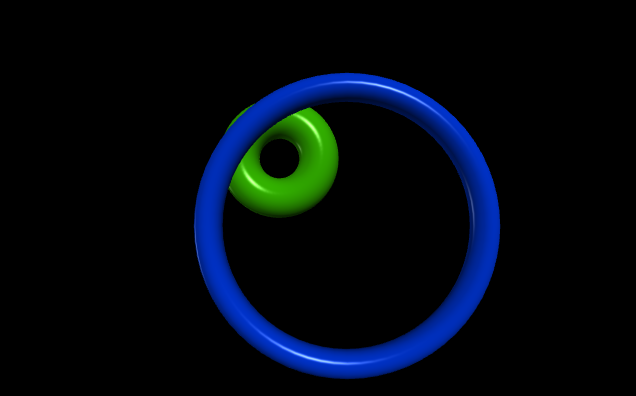GeeksforGeeks App
Open AppBrowser
Continue

# Making a ring with VPython

`VPython` makes it easy to create navigable 3D displays and animations, even for those with limited programming experience. Because it is based on Python, it also has much to offer for experienced programmers and researchers. `VPython` allows users to create objects such as spheres and cones in 3D space and displays these objects in a window. This makes it easy to create simple visualizations, allowing programmers to focus more on the computational aspect of their programs. The simplicity of `VPython` has made it a tool for the illustration of simple physics, especially in the educational environment.

Installation :

`pip install vpython`

A ring is a geometrical object in three-dimensional space that has a circular shape with hollow insides. We can generate a ring in `VPython` using the `ring()` method.

## ring() method

Syntax : ring(parameters)

Parameters :

• pos : It is the position of the center of the ring. Assign a vector containing 3 values, example pos = vector(0, 0, 0)
• axis : It is the axis of alignment of the ring. Assign a vector containing 3 values, example axis = vector(1, 2, 1)
• up : It is the orientation of the ring. Assign a vector containing 3 values, example up = vector(0, 1, 0)
• color : It is the color of the ring. Assign a vector containing 3 values, example color = vector(1, 1, 1) will give the color white
• opacity : It is the opacity of the ring. Assign a floating value in which 1 is the most opaque and 0 the least opaque, example opacity = 0.5
• shininess : It is the shininess of the ring. Assign a floating value in which 1 is the most shiny and 0 the least shiny, example shininess = 0.6
• emissive : It is the emissivity of the ring. Assign a boolean value in which True is emissive and False is not emissive, example emissivity = False
• texture : It is the texture of the ring. Assign the required texture from the textures class, example texture = textures.stucco
• radius : It is the radius of the ring. Assign a floating value, the default radius is 1, example radius = 5
• thickness : It is the radius of the cross section of the ring. Assign a floating value, the default thickness is 1/10 value of radius, example thickness = 8
• size : It is the size of the ring. Assign a vector containing 3 values representing the length, height and width respectively, example size = vector(1, 1, 1)

All the parameters are optional.

Example 1 :A ring with no parameters, all the parameters will have the default value.

 `# import the module``from` `vpython ``import` `*` `ring()`

Output :Example 2 :A ring using the parameters texture, axis and up.

 `# import the module``from` `vpython ``import` `*` `ring(texture ``=` `textures.wood,``     ``axis ``=` `vector(``0``, ``0``, ``1``),``     ``up ``=` `vector(``1``, ``0``, ``0``))`

Output :Example 3 :Displaying 2 rings to visualize the attributes pos, radius and thickness.

 `# import the module``from` `vpython ``import` `*`` ` `# the first ring``ring(pos ``=` `vector(``-``2``, ``2``, ``0``),``     ``axis ``=` `vector(``0``, ``0``, ``1``),``     ``radius ``=` `2``,``     ``thickness ``=` `1``,``     ``color ``=` `vector(``0.2``, ``0.7``, ``0``))`` ` `# the second ring``ring(pos ``=` `vector(``1``, ``-``1``, ``5``), ``     ``axis ``=` `vector(``0``, ``0``, ``1``),``     ``radius ``=` `5``,``     ``color ``=` `vector(``0``, ``0.2``, ``0.8``))`

Output :My Personal Notes arrow_drop_up